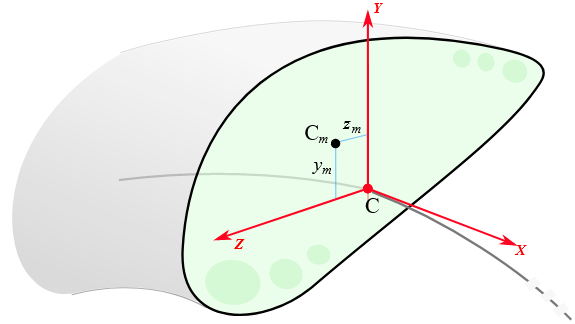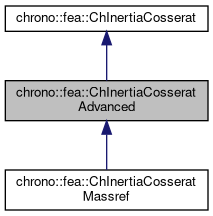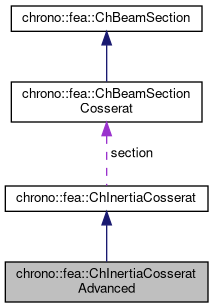## Description

Inertia properties of a beam of Cosserat type, not necessarily of uniform density, from the following information that allows the center of mass to be offset respect to the beam centerline:

• a mass per unit length
• offset of the center of mass Cm along Y Z section axes,
• Jyy Jzz Jzy moments of inertia computed in section reference Y Z, not rotated and origin in centerline The polar moment of area is automatically inferred via perpendicular axis theorem.#include <ChBeamSectionCosserat.h>

Inheritance diagram for chrono::fea::ChInertiaCosseratAdvanced:[legend]
Collaboration diagram for chrono::fea::ChInertiaCosseratAdvanced:[legend]

## Public Member Functions

ChInertiaCosseratAdvanced (double mu_density, double c_y, double c_z, double Jyy_moment, double Jzz_moment, double Jyz_moment)

ChInertiaCosseratAdvanced (double mu_density, double c_y, double c_z, ChVector<> Ivals)

virtual void ComputeInertiaMatrix (ChMatrixNM< double, 6, 6 > &M) override
Compute the 6x6 sectional inertia matrix, as in {x_momentum,w_momentum}=[Mi]{xvel,wvel} The matrix is computed in the material reference. More...

virtual void ComputeInertiaDampingMatrix (ChMatrixNM< double, 6, 6 > &Ri, const ChVector<> &mW) override
Compute the 6x6 sectional inertia damping matrix [Ri] (gyroscopic matrix damping), as in linearization dFi=[Mi]*d{xacc,wacc}+[Ri]*d{xvel,wvel}+[Ki]*d{pos,rot} The matrix is computed in the material reference. More...

virtual void ComputeInertiaStiffnessMatrix (ChMatrixNM< double, 6, 6 > &Ki, const ChVector<> &mWvel, const ChVector<> &mWacc, const ChVector<> &mXacc) override
Compute the 6x6 sectional inertia stiffness matrix [Ki^], as in linearization dFi=[Mi]*d{xacc,wacc}+[Ri]*d{xvel,wvel}+[Ki]*d{pos,rot} The matrix is computed in the material reference. More...

virtual void ComputeQuadraticTerms (ChVector<> &mF, ChVector<> &mT, const ChVector<> &mW) override
Compute the values of inertial force & torque depending on quadratic velocity terms, that is the gyroscopic torque w x [J]w and the centrifugal term (if center of mass is offset). More...

virtual double GetMassPerUnitLength () override
Get mass per unit length, ex.SI units [kg/m].

virtual void SetMassPerUnitLength (double mmu)
Set mass c, ex.SI units [kg/m]. More...

void SetCenterOfMass (double my, double mz)
"mass reference": set the displacement of the center of mass respect to the section centerline reference.

double GetCenterOfMassY ()

double GetCenterOfMassZ ()

virtual void SetInertiasPerUnitLength (double Jyy_moment, double Jzz_moment, double Jyz_moment)
Set inertia moments, assumed computed in the Y Z unrotated reference frame of the section at centerline, and defined as: $$J_{yy} = \int_\Omega \rho z^2 d\Omega$$, also Jyy = Mm(4,4) $$J_{zz} = \int_\Omega \rho y^2 d\Omega$$, also Jzz = Mm(5,5) $$J_{yz} = \int_\Omega \rho y z d\Omega$$, also Jyz = -Mm(4,5) = -Mm(5,4) Note that for an uniform density, these are also related to second moments of area as $$J_{yy} = \rho I_{yy}$$, $$J_{zz} = \rho I_{zz}$$. More...

virtual double GetInertiaJxxPerUnitLength ()
Get the Jxx component of the inertia per unit length (polar inertia), in the Y Z unrotated reference frame of the section at centerline. More...

virtual double GetInertiaJyyPerUnitLength ()
Get the Jyy component of the inertia per unit length, in the Y Z unrotated reference frame of the section at centerline, also Jyy = Mm(4,4)

virtual double GetInertiaJzzPerUnitLength ()
Get the Jzz component of the inertia per unit length, in the Y Z unrotated reference frame of the section at centerline, also Jzz = Mm(5,5)

virtual double GetInertiaJyzPerUnitLength ()
Get the Jyz off-diagonal component of the inertia per unit length, in the Y Z unrotated reference frame of the section at centerline. More...

virtual void SetMainInertiasInMassReference (double Jmyy, double Jmzz, double phi)
Set inertia moments, per unit length, as assumed computed in the Ym Zm "mass reference" frame, ie. More...

virtual void GetMainInertiasInMassReference (double &Jmyy, double &Jmzz, double &phi)
Get inertia moments, per unit length, as assumed computed in the Ym Zm "mass reference" frame, and the rotation phi of that frame, ie. More...Public Member Functions inherited from chrono::fea::ChInertiaCosserat
virtual void ComputeInertialForce (ChVector<> &mFi, ChVector<> &mTi, const ChVector<> &mWvel, const ChVector<> &mWacc, const ChVector<> &mXacc)
Compute the total inertial wrench, ie forces and torques (per unit length). More...

## Additional Inherited MembersPublic Attributes inherited from chrono::fea::ChInertiaCosserat
ChBeamSectionCosseratsection

bool compute_inertia_damping_matrix = true
Flag that turns on/off the computation of the [Ri] 'gyroscopic' inertial damping matrix. More...

bool compute_inertia_stiffness_matrix = true
Flag that turns on/off the computation of the [Ki] inertial stiffness matrix. More...

bool compute_Ri_Ki_by_num_diff = false
Flag for computing the Ri and Ki matrices via numerical differentiation even if an analytical expression is provided. More...

## ◆ ChInertiaCosseratAdvanced() [1/2]

 chrono::fea::ChInertiaCosseratAdvanced::ChInertiaCosseratAdvanced ( double mu_density, double c_y, double c_z, double Jyy_moment, double Jzz_moment, double Jyz_moment )
inline
Parameters
 mu_density mass per unit length [kg/m] c_y displacement of center of mass along Y c_z displacement of center of mass along Z Jyy_moment moment of inertia per unit length, about Y. Also Jyy= Mm(4,4) Jzz_moment moment of inertia per unit length, about Z. Also Jzz= Mm(5,5) Jyz_moment moment of inertia per unit length, about YZ (off diagonal term). Also Jyz= -Mm(4,5) = -Mm(5,4)

## ◆ ChInertiaCosseratAdvanced() [2/2]

 chrono::fea::ChInertiaCosseratAdvanced::ChInertiaCosseratAdvanced ( double mu_density, double c_y, double c_z, ChVector<> Ivals )
inline
Parameters
 mu_density mass per unit length [kg/m] c_y displacement of center of mass along Y c_z displacement of center of mass along Z

## Member Function Documentation

 void chrono::fea::ChInertiaCosseratAdvanced::ComputeInertiaDampingMatrix ( ChMatrixNM< double, 6, 6 > & Ri, const ChVector<> & mW )
overridevirtual

Compute the 6x6 sectional inertia damping matrix [Ri] (gyroscopic matrix damping), as in linearization dFi=[Mi]*d{xacc,wacc}+[Ri]*d{xvel,wvel}+[Ki]*d{pos,rot} The matrix is computed in the material reference.

Parameters
 Ri 6x6 sectional inertial-damping (gyroscopic damping) matrix values here mW current angular velocity of section, in material frame

Reimplemented from chrono::fea::ChInertiaCosserat.

## ◆ ComputeInertiaMatrix()

 void chrono::fea::ChInertiaCosseratAdvanced::ComputeInertiaMatrix ( ChMatrixNM< double, 6, 6 > & M )
overridevirtual

Compute the 6x6 sectional inertia matrix, as in {x_momentum,w_momentum}=[Mi]{xvel,wvel} The matrix is computed in the material reference.

Parameters
 M 6x6 sectional mass matrix values here

Implements chrono::fea::ChInertiaCosserat.

## ◆ ComputeInertiaStiffnessMatrix()

 void chrono::fea::ChInertiaCosseratAdvanced::ComputeInertiaStiffnessMatrix ( ChMatrixNM< double, 6, 6 > & Ki, const ChVector<> & mWvel, const ChVector<> & mWacc, const ChVector<> & mXacc )
overridevirtual

Compute the 6x6 sectional inertia stiffness matrix [Ki^], as in linearization dFi=[Mi]*d{xacc,wacc}+[Ri]*d{xvel,wvel}+[Ki]*d{pos,rot} The matrix is computed in the material reference.

NOTE the matrix already contains the 'geometric' stiffness, so it transforms to absolute transl/local rot just like [Mi] and [Ri]: [Ki]_al =[R,0;0,I]*[Ki^]*[R',0;0,I'] , with [Ki^]=([Ki]+[0,f~';0,0]) for f=current force part of inertial forces.

Parameters
 Ki 6x6 sectional inertial-stiffness matrix [Ki^] values here mWvel current angular velocity of section, in material frame mWacc current angular acceleration of section, in material frame mXacc current acceleration of section, in material frame (not absolute!)

Reimplemented from chrono::fea::ChInertiaCosserat.

 void chrono::fea::ChInertiaCosseratAdvanced::ComputeQuadraticTerms ( ChVector<> & mF, ChVector<> & mT, const ChVector<> & mW )
overridevirtual

Compute the values of inertial force & torque depending on quadratic velocity terms, that is the gyroscopic torque w x [J]w and the centrifugal term (if center of mass is offset).

All terms expressed in the material reference, ie. the reference in the centerline of the section.

Parameters
 mF centrifugal term (if any) returned here mT gyroscopic term returned here mW current angular velocity of section, in material frame

Implements chrono::fea::ChInertiaCosserat.

## ◆ GetInertiaJxxPerUnitLength()

 virtual double chrono::fea::ChInertiaCosseratAdvanced::GetInertiaJxxPerUnitLength ( )
inlinevirtual

Get the Jxx component of the inertia per unit length (polar inertia), in the Y Z unrotated reference frame of the section at centerline.

Note: it automatically follows Jxx=Jyy+Jzz for the polar theorem.

## ◆ GetInertiaJyzPerUnitLength()

 virtual double chrono::fea::ChInertiaCosseratAdvanced::GetInertiaJyzPerUnitLength ( )
inlinevirtual

Get the Jyz off-diagonal component of the inertia per unit length, in the Y Z unrotated reference frame of the section at centerline.

Also Jyz = -Mm(4,5) = -Mm(5,4)

## ◆ GetMainInertiasInMassReference()

 void chrono::fea::ChInertiaCosseratAdvanced::GetMainInertiasInMassReference ( double & Jmyy, double & Jmzz, double & phi )
virtual

Get inertia moments, per unit length, as assumed computed in the Ym Zm "mass reference" frame, and the rotation phi of that frame, ie.

inertias centered at the center of mass and rotated by phi angle to match the main axes of inertia: $$Jm_{yy} = \int_\Omega \rho z_{m}^2 d\Omega$$, $$Jm_{zz} = \int_\Omega \rho y_{m}^2 d\Omega$$. Assuming the center of mass is already set.

Reimplemented in chrono::fea::ChInertiaCosseratMassref.

## ◆ SetInertiasPerUnitLength()

 void chrono::fea::ChInertiaCosseratAdvanced::SetInertiasPerUnitLength ( double Jyy_moment, double Jzz_moment, double Jyz_moment )
virtual

Set inertia moments, assumed computed in the Y Z unrotated reference frame of the section at centerline, and defined as: $$J_{yy} = \int_\Omega \rho z^2 d\Omega$$, also Jyy = Mm(4,4) $$J_{zz} = \int_\Omega \rho y^2 d\Omega$$, also Jzz = Mm(5,5) $$J_{yz} = \int_\Omega \rho y z d\Omega$$, also Jyz = -Mm(4,5) = -Mm(5,4) Note that for an uniform density, these are also related to second moments of area as $$J_{yy} = \rho I_{yy}$$, $$J_{zz} = \rho I_{zz}$$.

Note also that $$J_{xy} = J_{xz} = J_{yx} = J_{zx} = 0$$ anyway. Note also that $$J_{xy}$$ does not need to be input, as automatically computed via $$J_{xx} = J_{yy} +J_{zz}$$ for the polar theorem.

Reimplemented in chrono::fea::ChInertiaCosseratMassref.

## ◆ SetMainInertiasInMassReference()

 void chrono::fea::ChInertiaCosseratAdvanced::SetMainInertiasInMassReference ( double Jmyy, double Jmzz, double phi )
virtual

Set inertia moments, per unit length, as assumed computed in the Ym Zm "mass reference" frame, ie.

centered at the center of mass and rotated by phi angle to match the main axes of inertia: $$Jm_{yy} = \int_\Omega \rho z_{m}^2 d\Omega$$, $$Jm_{zz} = \int_\Omega \rho y_{m}^2 d\Omega$$. Assuming the center of mass is already set.

Reimplemented in chrono::fea::ChInertiaCosseratMassref.

## ◆ SetMassPerUnitLength()

 virtual void chrono::fea::ChInertiaCosseratAdvanced::SetMassPerUnitLength ( double mmu )
inlinevirtual

Set mass c, ex.SI units [kg/m].

Note that for uniform volumetric density $$\rho$$, and area $$A$$, this is also $$\mu = \rho A$$.

The documentation for this class was generated from the following files:
• /builds/uwsbel/chrono/src/chrono/fea/ChBeamSectionCosserat.h
• /builds/uwsbel/chrono/src/chrono/fea/ChBeamSectionCosserat.cpp